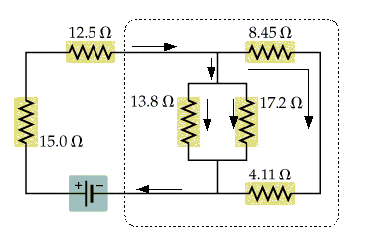# Need help with a circuit problem? (Picture attached)

**PICTURE ATTACHED AT BOTTOM**

## Homework Statement

The current in the 13.8-Ω resistor in the attached picture is .795 A. Find the current in the other resistors in the circuit.

## Homework Equations

V=RI

In a series circuit:
V of Circuit = V1 + V2 + V3 +...Vn
I of Circuit = I1 = I2 = I3 = ...In
R of Circuit = R1 + R2 + R3 +... Rn

In a parallel circuit:
V of Circuit = V1 = V2 = V3 = ... Vn
I of Circuit = I1 + I2 + I3 +... In
1/R of Circuit = 1/(R1) + 1/(R2) + 1/(R3) +... 1/(Rn)

## The Attempt at a Solution

For the 13.8-Ω resistor, I found that Voltage = 10.971 by using V=IR
- Because V of Circuit = (V1 = V2 = V3 = ... Vn), the Voltage for the 17.2-Ω resistor is also 10.971
--Based on this, the Current for the 17.2-Ω resistor is .638:
V=IR
I=V/R
I=(10.971)/(17.2)
I=.638

After this, I proceeded to find the equivalent resistance for the entire circuit.

To find this, I separated the circuits into groups.

Group 1= the 15-Ω and 12.5-Ω resistors (they are in series).
Group 2= the 13.8-Ω and 17.2-Ω resistors (they are parallel).
Group 3= the 8.45-Ω and 4.11-Ω resistors (they are in series).

When solved for, the equivalent resistance for each group is as follows:

Group 1= 27.5-Ω
Group 2= 7.66-Ω
Group 3= 12.56-Ω

Note that Groups 2 and 3 are now parallel. We will combine them into Group 4.

Group 4 Resistance = 4.75-Ω

Now we only have Groups 1 and 4 left in series. When combined, we will get the net resistance for the entire Circuit. This works out to be 32.56-Ω

.....So that's everything I could find, which is decent, but doesn't come anywhere near answering the question. I know I must be missing something somewhere.

How do I figure out the individual currents for each resistor with the information I currently have?

#### Attachments

•Walker.21.44.jpg
14.1 KB · Views: 745

## Answers and Replies

gneill
Mentor
**PICTURE ATTACHED AT BOTTOM**

## Homework Statement

The current in the 13.8-Ω resistor in the attached picture is .795 A. Find the current in the other resistors in the circuit.

## Homework Equations

V=RI

In a series circuit:
V of Circuit = V1 + V2 + V3 +...Vn
I of Circuit = I1 = I2 = I3 = ...In
R of Circuit = R1 + R2 + R3 +... Rn

In a parallel circuit:
V of Circuit = V1 = V2 = V3 = ... Vn
I of Circuit = I1 + I2 + I3 +... In
1/R of Circuit = 1/(R1) + 1/(R2) + 1/(R3) +... 1/(Rn)

## The Attempt at a Solution

For the 13.8-Ω resistor, I found that Voltage = 10.971 by using V=IR
- Because V of Circuit = (V1 = V2 = V3 = ... Vn), the Voltage for the 17.2-Ω resistor is also 10.971
--Based on this, the Current for the 17.2-Ω resistor is .638:
V=IR
I=V/R
I=(10.971)/(17.2)
I=.638

After this, I proceeded to find the equivalent resistance for the entire circuit.

<snip!>

You're doing so well using the voltage across the 13.8Ω resistor, why not continue? The same voltage must be across the series connected 8.45Ω + 4.11Ω resistors. So what's the current through them?

What then is the total current for the subcircuit?#### Attachments

Ah, of course!

Okay, so based on that...

Current of the 8.45 Ω resistor should be (10.971)/(8.45) = 1.3 A

and the 4.11 Ω will be (10.971)/(4.11) = 2.67 A

So the total for the subcircuit will be

.795 A
+.638 A
+1.3 A
+2.67 A

= 5.403 A

....
Would that be correct?

gneill
Mentor
Ah, of course!

Okay, so based on that...

Current of the 8.45 Ω resistor should be (10.971)/(8.45) = 1.3 A

and the 4.11 Ω will be (10.971)/(4.11) = 2.67 A

So the total for the subcircuit will be

.795 A
+.638 A
+1.3 A
+2.67 A

= 5.403 A

....
Would that be correct?

No, not quite. The 8.45 and 4.11 resistors are in series. How do you combine series resistances?

No, not quite. The 8.45 and 4.11 resistors are in series. How do you combine series resistances?

So would it be

(10.971)/(8.45+4.11) = .873 A

And then the subcircuit would work out as

.873 A
+ .795 A
+ .638 A

= 2.3 A

gneill
Mentor
So would it be

(10.971)/(8.45+4.11) = .873 A

And then the subcircuit would work out as

.873 A
+ .795 A
+ .638 A

= 2.3 A

Yessir. That looks good. You should now be in a position to state the current through every resistor in the circuit.

Alrighty, here are my final results. Everything seems to check out:

13 Ω = .795 A
17.2 Ω = .638 A
8.41 Ω = .87 A
4.11 Ω = .87 A
15 Ω = 2.3 A
12.56 Ω = 2.3 A

gneill
Mentor
Alrighty, here are my final results. Everything seems to check out:

13 Ω = .795 A
17.2 Ω = .638 A
8.41 Ω = .87 A
4.11 Ω = .87 A
15 Ω = 2.3 A
12.56 Ω = 2.3 A

Yup.

thanks for all the help.